# Inter Maths solutions for Applications of Derivatives

Intermediate Mathematics 1B Applications of Derivatives exercise 10(g) textbook solutions are given.

Study the lesson Applications of Derivatives very well.

Practice the example problems and solutions given in the textbook.

Observe the given below solutions and try them in your own method.

You can also see

Inter Maths 1A textbook solutions

Inter Maths 1B textbook solutions

Inter Maths IIA textbook solutions

Inter Maths IIB textbook solutions

Applications of Derivatives

Exercise 10(a)

Exercise 10(b)

Exercise 10(c)

Exercise 10(d)

Exercise 10(e)

Exercise 10(f)

Exercise 10(g)

Exercise 10(h)

M

## Exercise 10(g) Solutions Applications of Derivatives Inter Maths 1B

Chapter – 10 Applications of Derivatives

Exercise 10(g)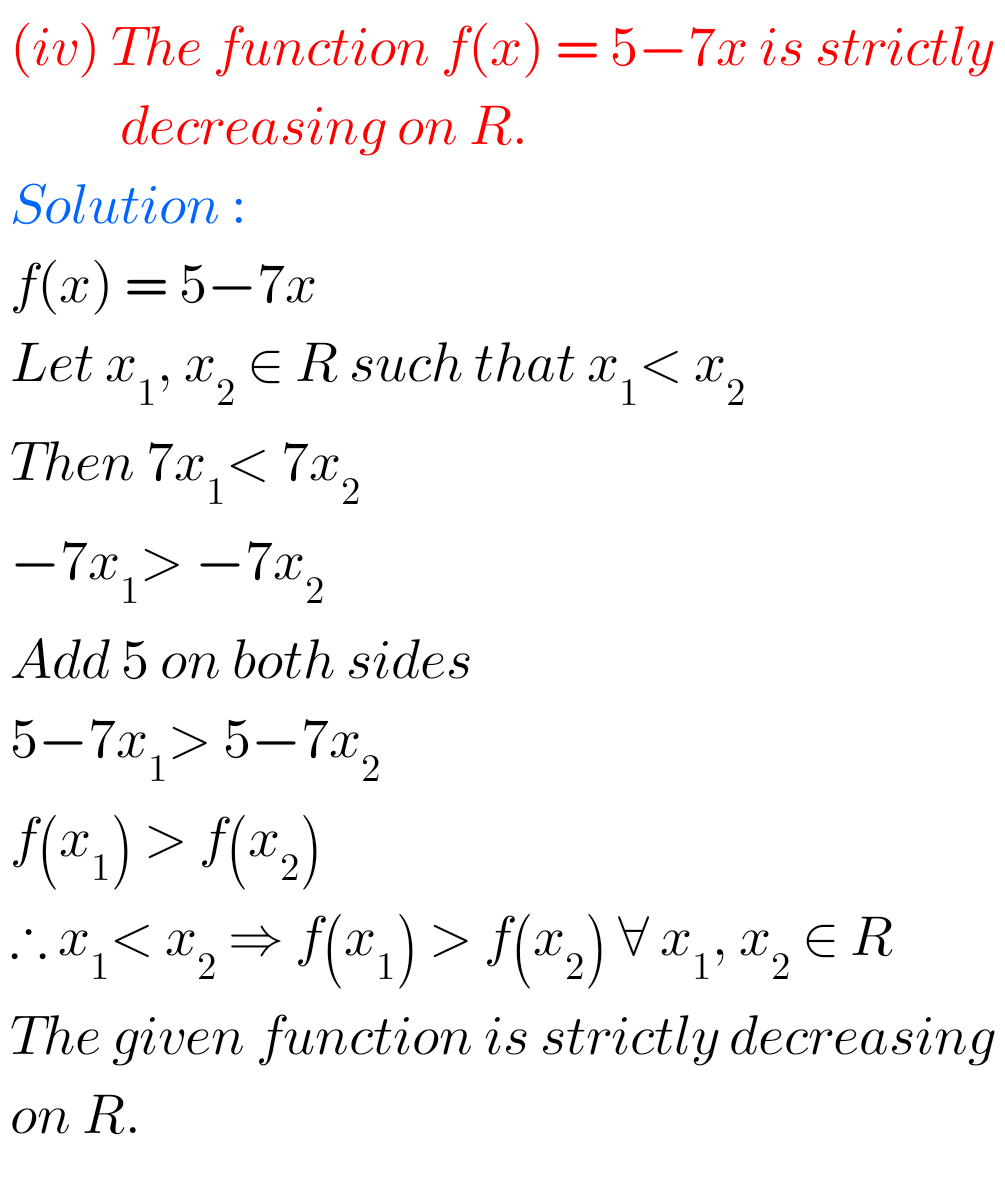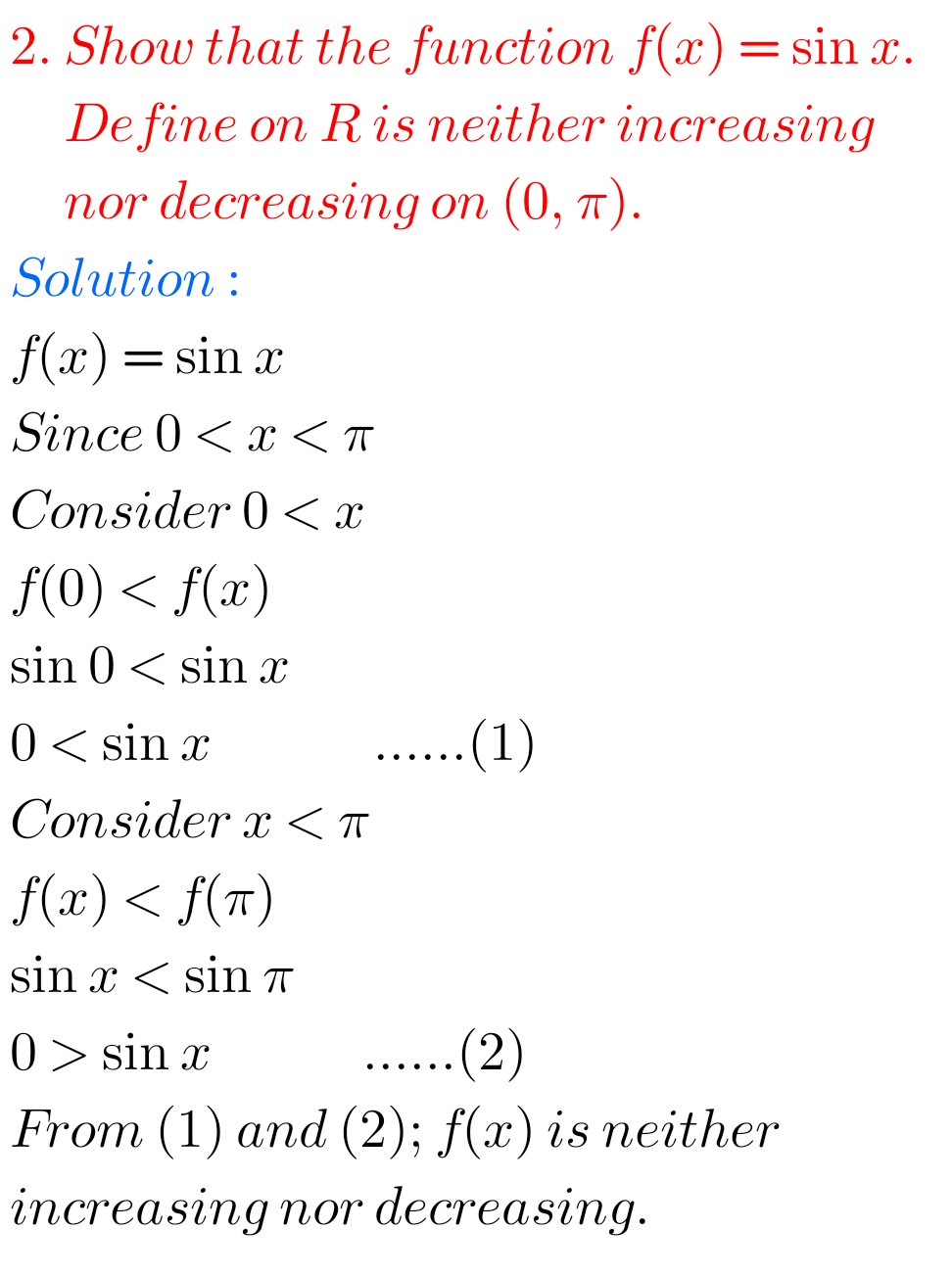## Maths 1B Solutions for Applications of Derivatives Inter exercise 10(g)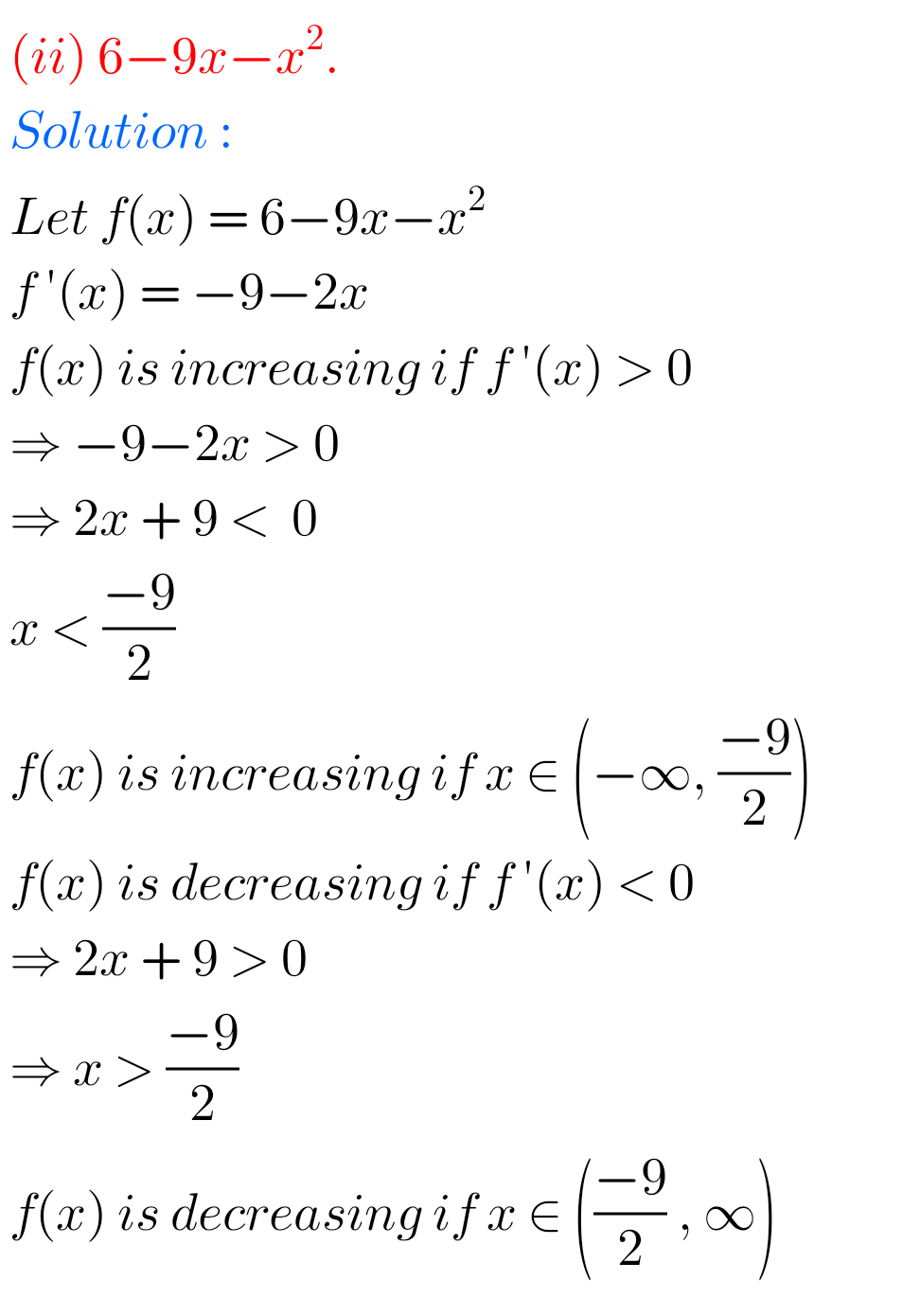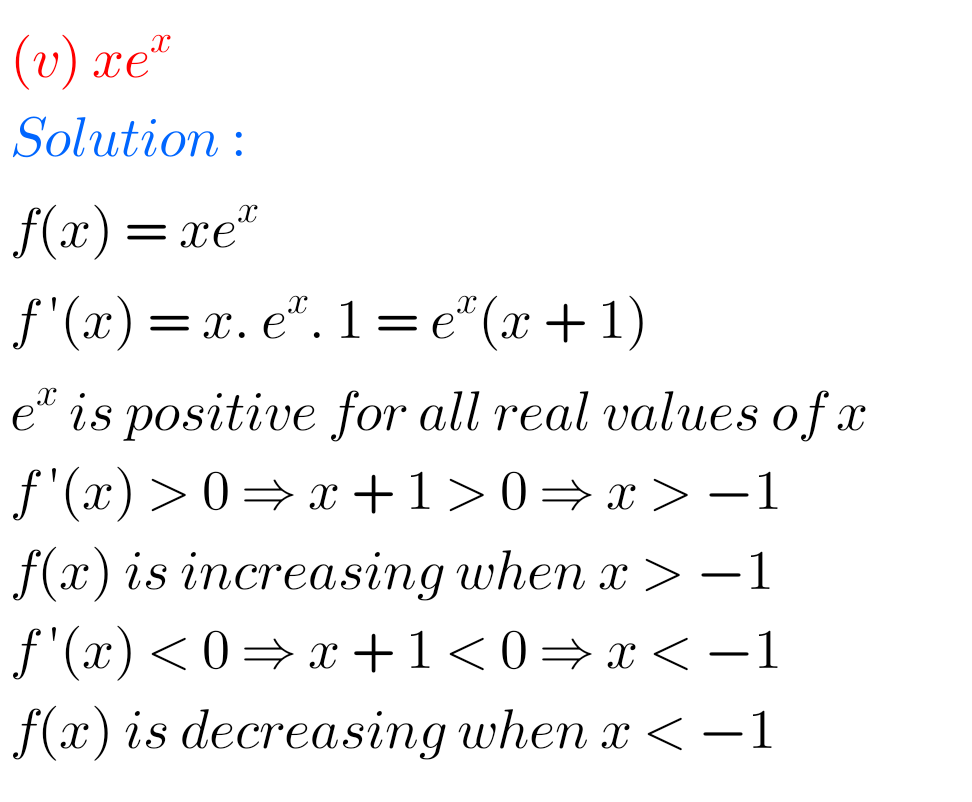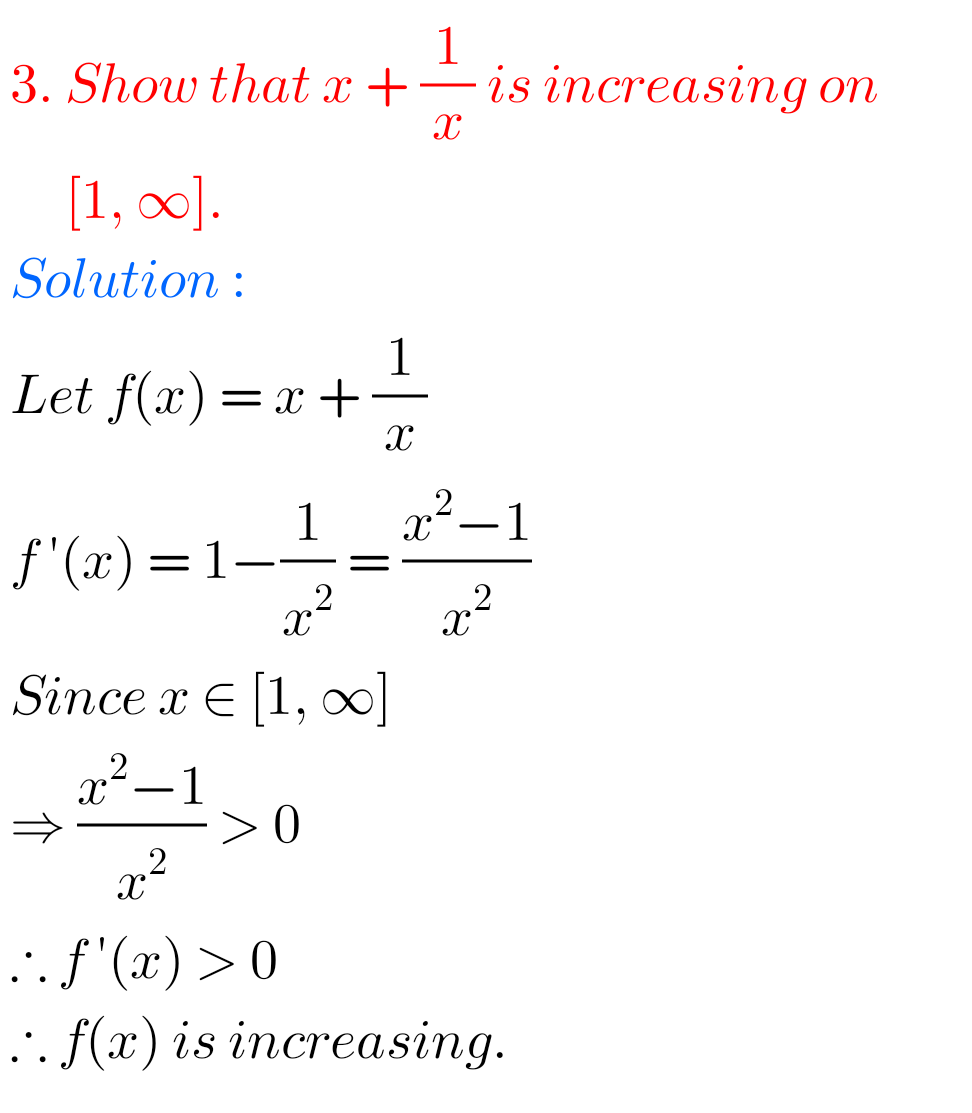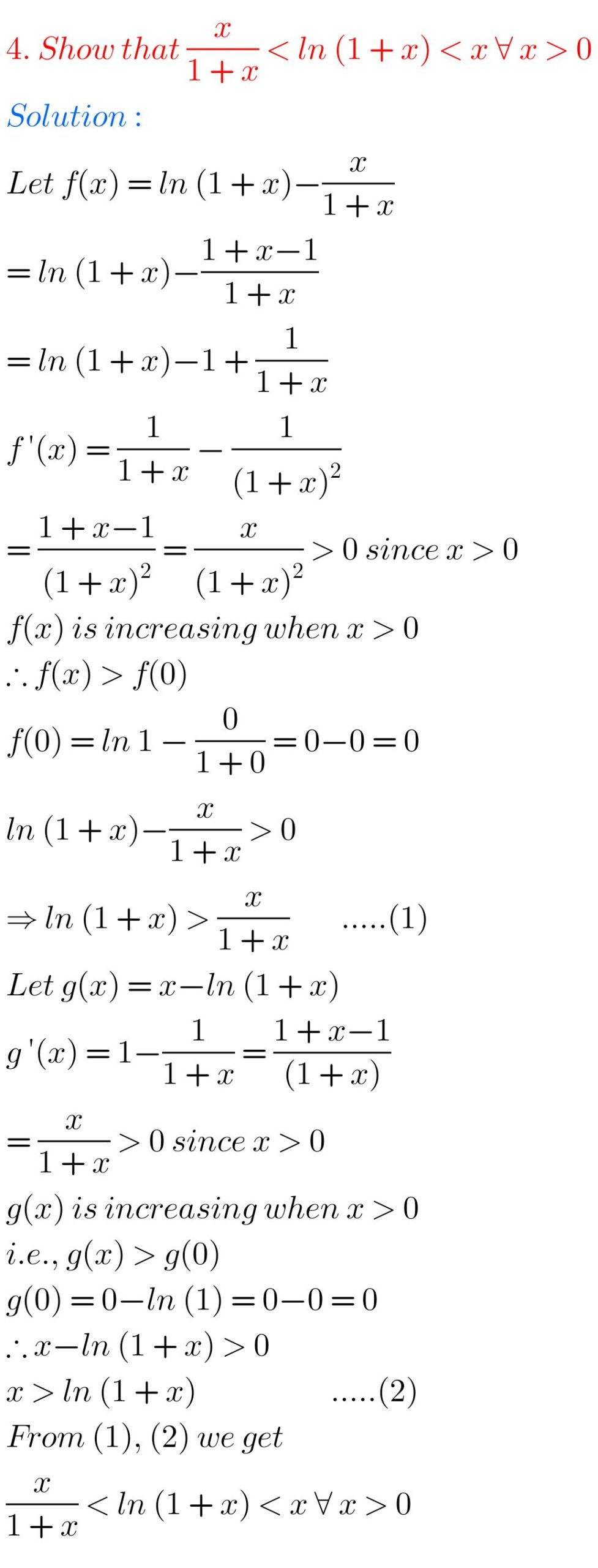## Solutions for exercise 10(g) Inter First year Maths 1B Applications of Derivatives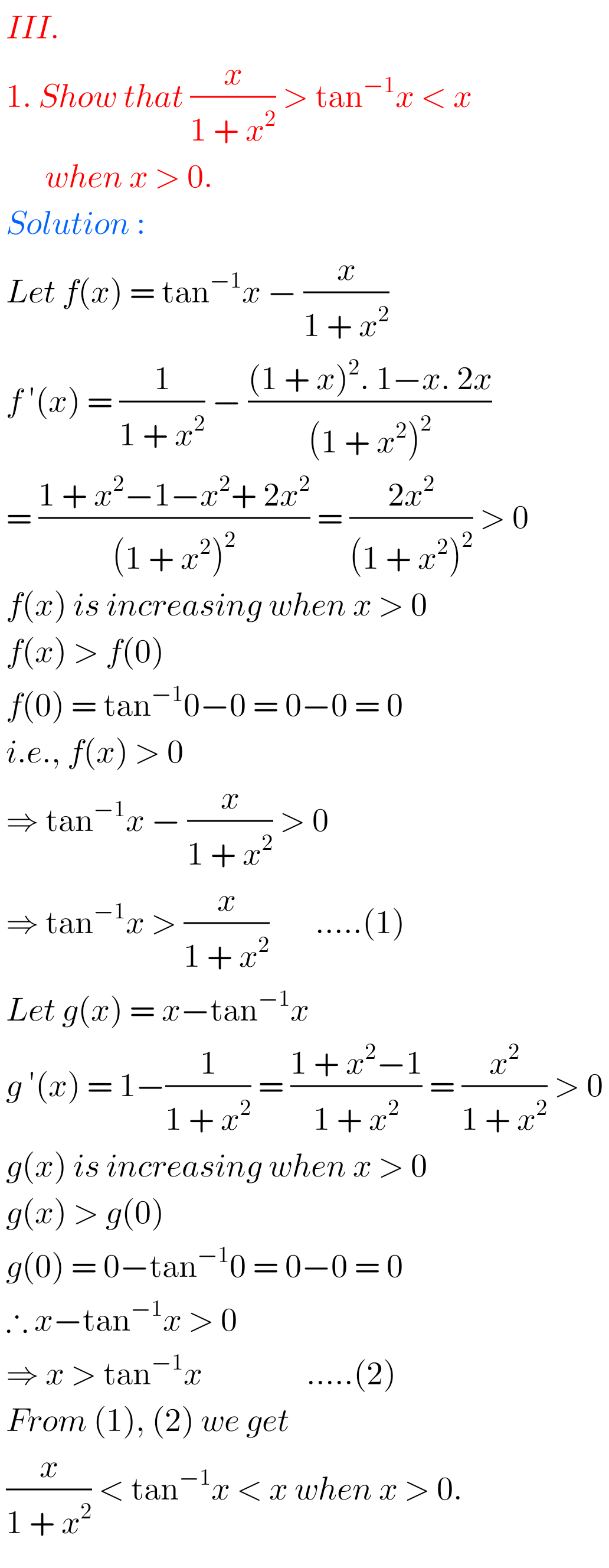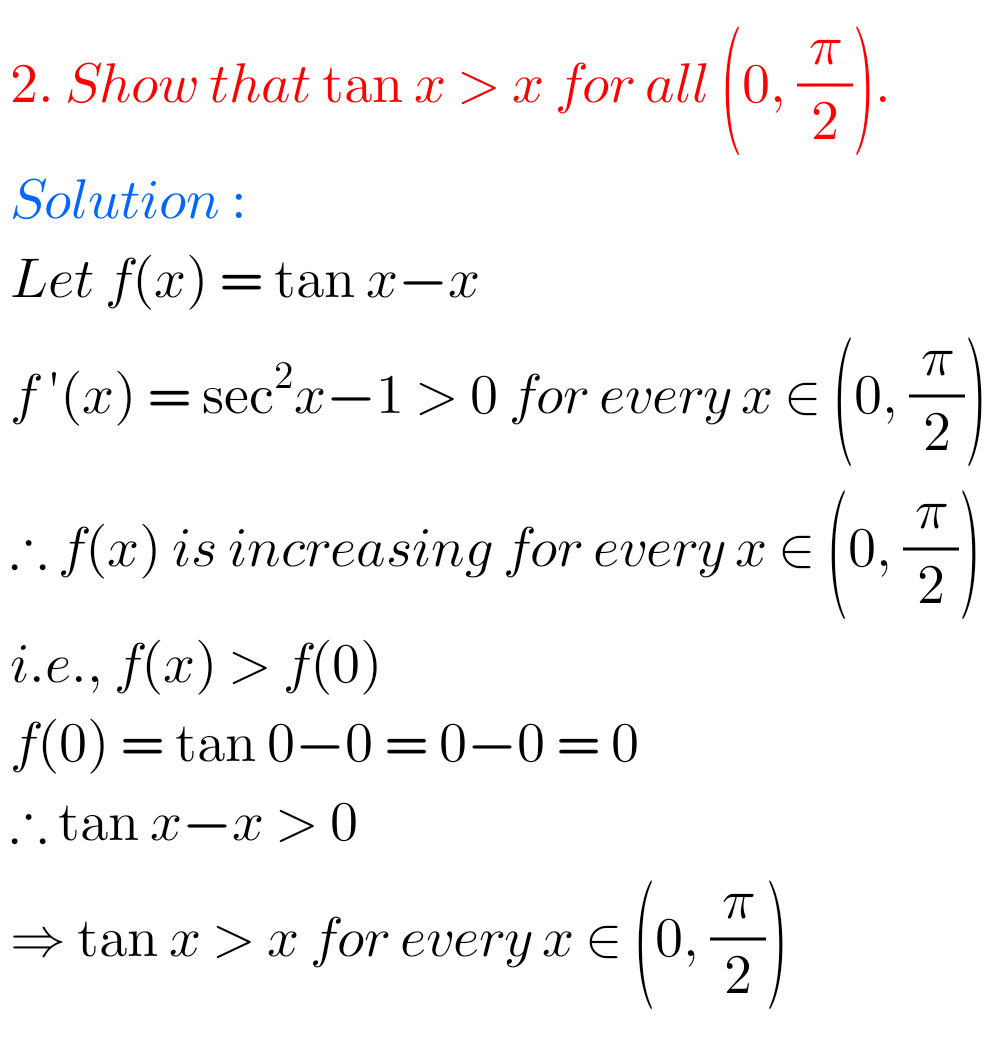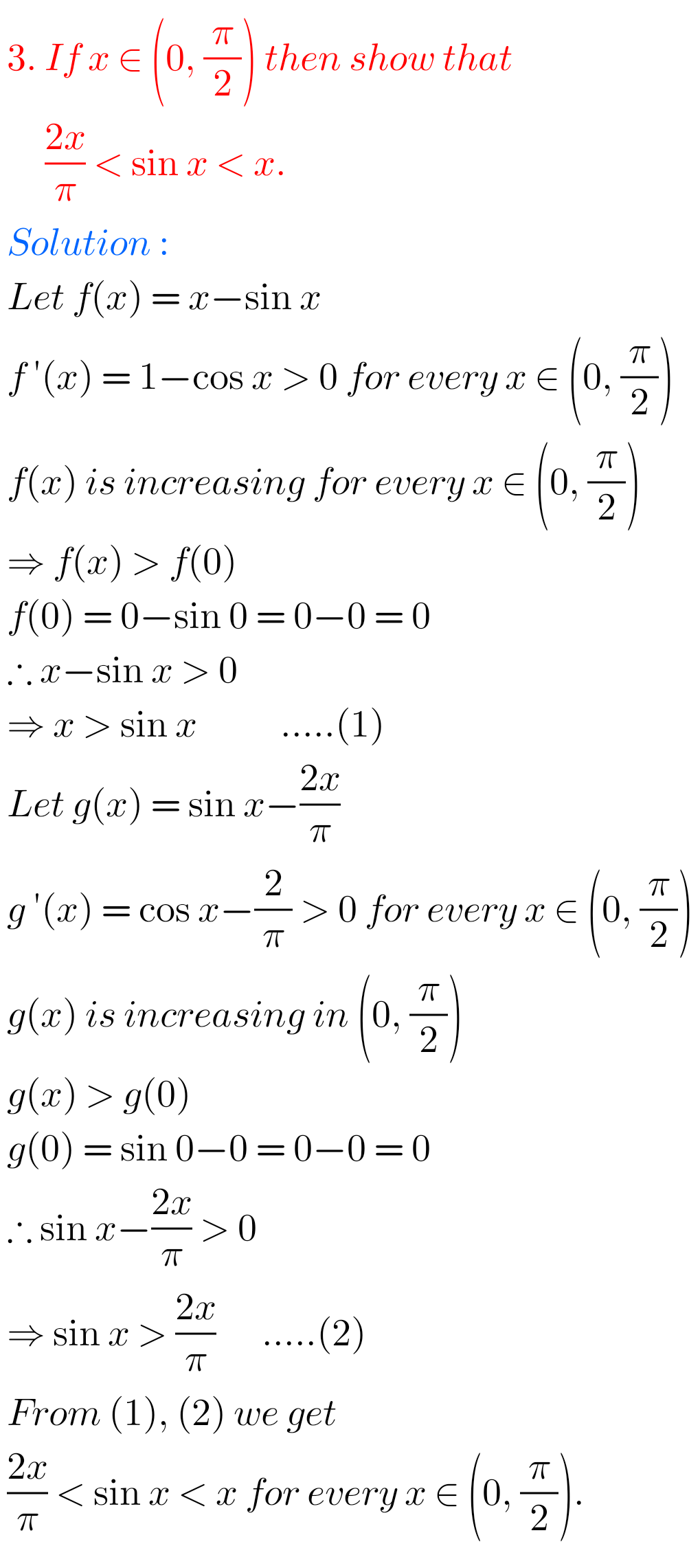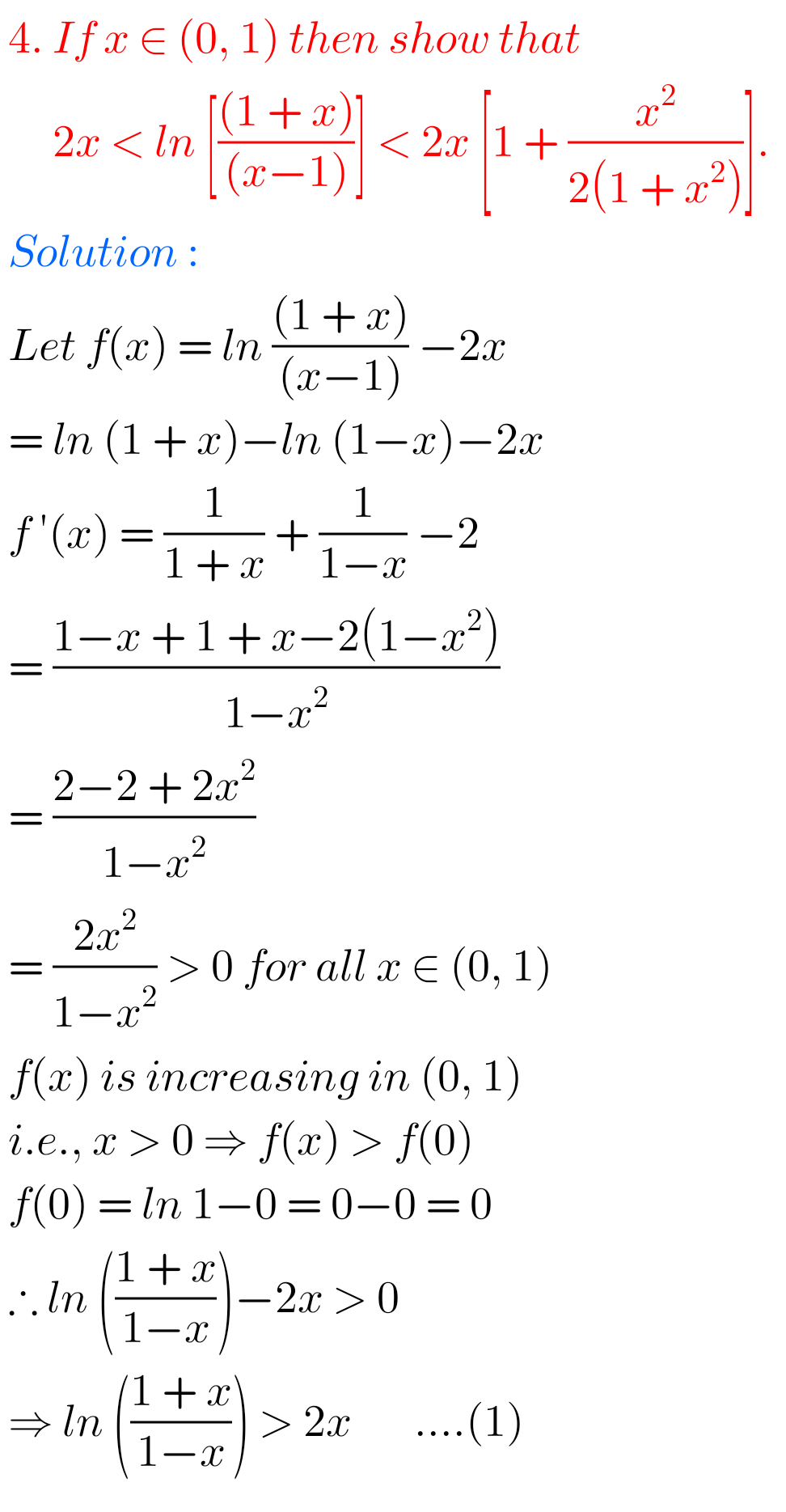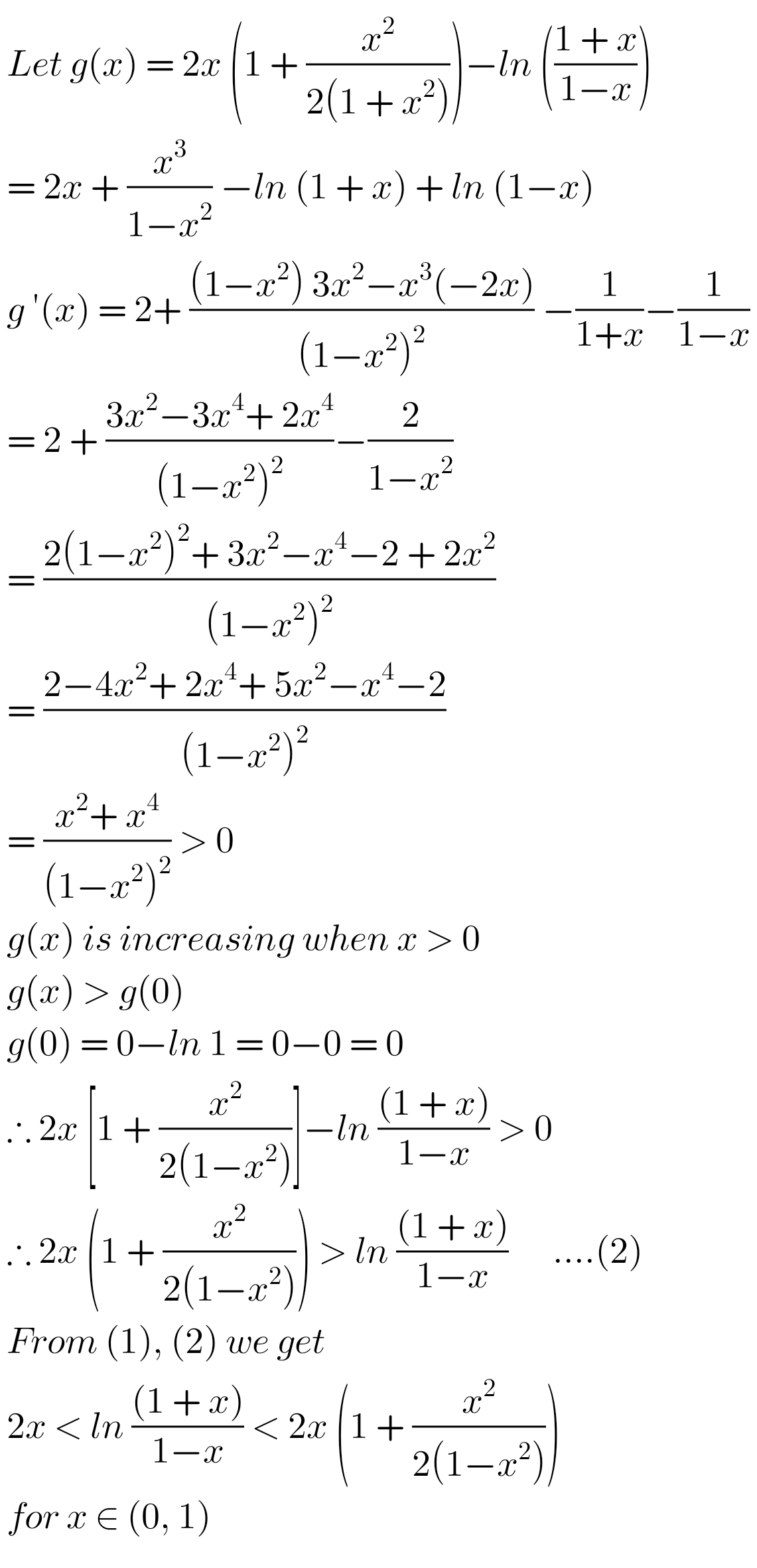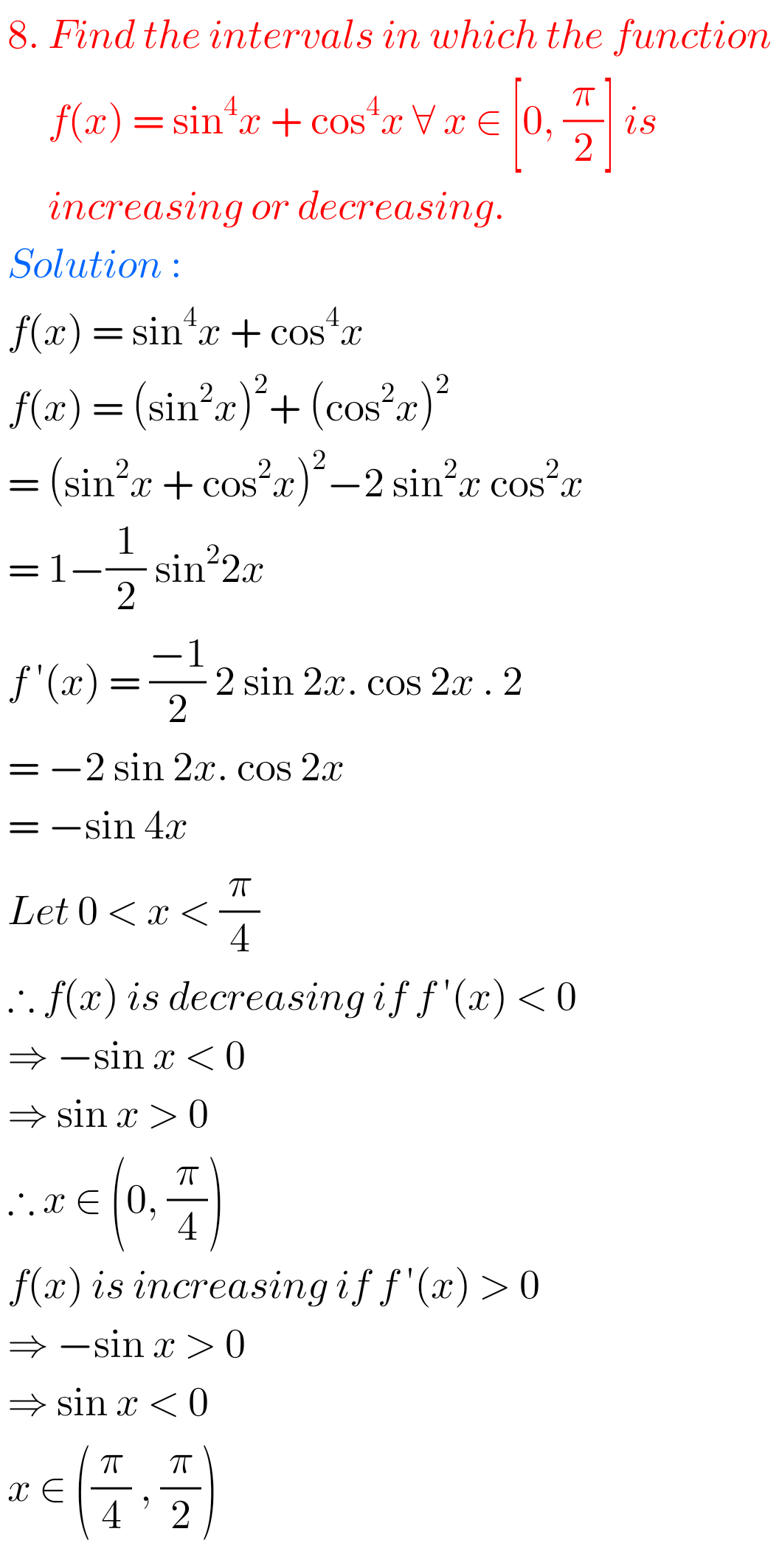Note : Observe the solutions and try them in your own method.